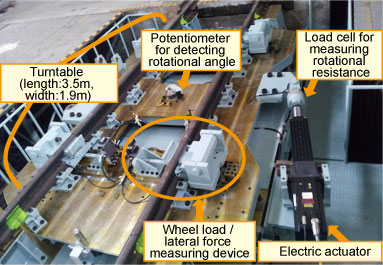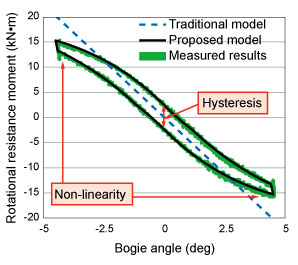# 8. Method for evaluating bogie rotational characteristics

• A device was developed for testing bogie rotational characteristics.
• By measuring the resistance moment of a bogie, it is possible to obtain a more accurate evaluation of its characteristics when running through a curve.

A bogie follows a curve as it runs through it and the bogie angle (mutual yawing angle between car body and bogie) produced causes the secondary suspension and dampers to exert an opposing force. This rotation resistance moment of the bogie is one of the sources of lateral force exerted when running through a curve, it is therefore important to gain clear insight into this phenomenon in order to be able to evaluate the running behaviour of a railway vehicle in a curve.

Usually the rotation resistance moment of the bogie is estimated by combining the results of tests on individual components, e.g., air springs and dampers.

In order to obtain more accurate understanding of this phenomenon, a test was set up using a bogie equipped with air springs and dampers to which a carbody was added to replicate a vehicle load, in order to reproduce conditions as close as possible to a real situation (Fig.1).

The experimental set up for this research allows simultaneous measurement of the rotational resistance moment of the bogie, as well as other important elements for understanding vehicle behaviour during rotation, i.e. wheel load, lateral force, bogie displacement and forces in three directions exerted by the air springs and also allows measurement of the bogie inertia radius, thanks to multiple rotation modes. In addition, the set up complies with European Rail vehicle testing standards.

The rotational resistance moment of a bogie on a real vehicle was measured and some characteristics such as, non-linearity at large bogie angles, and hysteresis were observed(Fig.2). The rotational resistance moment of the bogie was also measured for cases where the air-springs were deflated or with yaw dampers in place. The data obtained through these experiments will be adapted for use in a simulation model and for creating a lateral force estimation formula in order to produce a rotational resistance model which is as close as possible to the real situation. This will hopefully lead to more accurate assessment of characteristics when running through a curve.

•Fig.1 Set up for examining bogie rotational characteristics
•Fig.2 Proposed model for finding rotational
resistance moment of bogie